## CODE4LIB@LISTS.CLIR.ORG

#### View:

 Message:[First|Previous|Next|Last] By Topic:[First|Previous|Next|Last] By Author:[First|Previous|Next|Last] Font: Proportional Font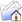LISTSERV Archives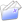CODE4LIB HomeCODE4LIB March 2016

Subject:Re: Validating MARC records

From:Date:Mon, 28 Mar 2016 12:51:28 -0400

Content-Type:multipart/mixed

Parts/Attachments: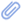text/plain (42 lines) , marcdiag (436 lines)
 ```On Mon, Mar 28, 2016 at 02:01:42PM +0000, Jiao, Dazhi wrote: > At IU we are running our OPAC using Blacklight. From time to time > there are some catalog errors that would cause errors in our custom > code to extract fields from the MARC records. For example, sometimes a > subfield may unexpectedly appear in a field, or an expected subfield > is accidentally named to another subfield. > > While we can catch these errors in our code, we’d also like to be able > to discover them and notify the catalogers before the records are > exposed in the discover layer. I wonder if anyone here has experiences > with some MARC validation tool for this purpose? Are you talking about low-level structural problems, like the record length field (Ldr/00-05) not matching the actual record length? Or high-level errors, like a 245 field without a subfield \$a or an invalid country code? If it's the latter, Bryan Baldus wrote a Perl module (MARC::Lint) that looks pretty comprehensive: https://metacpan.org/release/MARC-Lint It includes a script (marclint) that you can use directly from the command line. If it's the former, I've attached a Perl script I wrote (marcdiag) that catches most low-level errors. It only has one dependency -- Getopt::Long, which is pretty standard but not part of a core Perl installation. Paul. -- Paul Hoffman <[log in to unmask]> Systems Librarian Fenway Libraries Online c/o Wentworth Institute of Technology 550 Huntington Ave. Boston, MA 02115 (617) 442-2384 (FLO main number) #!/m1/shared/bin/perl use strict; use warnings; use bytes; use Getopt::Long     qw(:config posix_default gnu_compat require_order bundling no_ignore_case); use constant RECORD_TERMINATOR => "\x1d"; use constant FIELD_TERMINATOR => "\x1e"; use constant SUBFIELD_DELIMITER => "\x1f"; sub emit; sub summarize; sub record; sub fatal; sub info; sub error; sub warning; sub ok; sub fail; my \$rx_record_terminator = qr/\x1d/; my \$rx_field_terminator = qr/\x1e/; my \$rx_subfield_delimiter = qr/\x1f/; my \$rx_struc_char = qr/[\x1d-\x1f]/; my \$rx_nonstruc_char = qr/[^\x1d-\x1f]/; my %ecode2format = (     L05 => "Unrecognized record status: %s",     L06 => "Unrecognized record type: %s",     L07 => "Unrecognized bib level: %s",     L08 => "Unrecognized control type: %s",     L09 => "Unrecognized character encoding: %s",     L10 => "Invalid indicator count: %s",     L11 => "Invalid subfield code count: %s",     L17 => "Unrecognized encoding level: %s",     L18 => "Unrecognized cataloging form: %s",     M18 => "Unrecognized item info: %s",     L19 => "Unrecognized multipart resource record level: %s",     L20 => "Invalid length-of-field length: %s",     L21 => "Invalid length-of-offset length: %s",     L22 => "Invalid length-of-impldef length: %s",     L23 => "Invalid undefined value in leader: %s",     DLN => "Directory length: not a multiple of 12 bytes",     DNT => "Directory not terminated",     EFT => "Embedded field terminator in field %s",     ERT => "Embedded record terminator in field %s",     ETG => "Invalid field tag %s",     ESD => "Subfield delimiter in control field %s",     IIN => "Invalid indicator in field %s",     ISI => "Invalid subfield identifier %s in field %s",     IUT => "Invalid UTF-8 in field %s",     JAB => "Junk at beginning of field %s",     JAE => "Junk at end of field %s",     MSI => "Missing subfield identifier in field %s",     NSF => "Empty subfield %s in field %s",     NWF => "Not a USMARC record: pathological leader",     RLN => "Record length doesn't match the length encoded in the leader",     TRU => "Truncated field %s",     UFD => "Unterminated field %s", ); my %rstat2label = (     'a' => 'increase in encoding level',     'c' => 'corrected or revised',     'd' => 'deleted',     'n' => 'new',     'p' => 'increase in encoding level from prepublication', ); my (\$show_error_details, \$verbose, \$parseable, \$terse, \$quiet, \$check_utf8, \$strict); my \$summarize = 1; my %ignore; my (%err, %errmsg, %enc, %bad); my %rstat = ('c' => 0, 'd' => 0, 'n' => 0); my \$max_errs_in_record = 1; my \$max_errs_total = 1<<31; GetOptions(     'h' => \&usage,     'l' => \&list_codes,     'r=i' => \\$max_errs_in_record,     't=i' => \\$max_errs_total,     'a' => sub { \$max_errs_total = \$max_errs_in_record = 1 << 31 },     '1' => sub { \$max_errs_total = \$max_errs_in_record = 1 },     'u' => \\$check_utf8,     's' => \\$strict,     'e' => \\$show_error_details,     'x=s' => sub { \$ignore{\$_} = 1 for split /,/, \$_ },     'v' => \\$verbose,     'E' => \\$terse,     'n' => sub { \$summarize = 0 },     'p' => \\$parseable,     'q' => \\$quiet, ) or usage(); my (\$file, \$fh); if (@ARGV == 1) {     \$file = shift @ARGV;     open \$fh, '<', \$file         or fatal "Can't open input file \$file: \$!"; } elsif (@ARGV == 0) {     \$file = '';     \$fh = \*STDIN; } else {     usage(); } binmode \$fh; binmode STDOUT; my (\$n, \$rtype_bib, \$rtype_mfhd, \$rtype_auth, \$rtype_other) = (0, 0, 0, 0, 0); my \$skipped = 0; my \$byte_pos = 0; my \$bibid; my \$printed; my \$errs_total = 0; my \$errs_in_record = 0; \$/ = RECORD_TERMINATOR; RECORD: while (defined(my \$rec = <\$fh>)) {     \$n++;     \$errs_in_record = 0;     undef \$printed;     eval {         my (\$rlen, \$rstat, \$rtype, \$blvl, \$ctype, \$enc, \$icount, \$scount, \$baddr, \$elvl, \$cform, \$mrrl, \$loflen, \$ofslen, \$implen, \$undef) =         (             \$rec =~ m{                 \A                         # bytes description                         # ----- -------------------------------------------                 (\d{5}) # 00-04 Rec length                 (.) # 05 Record status                 (.) # 06 Type of record                 (.) # 07 Bibliographic Level                 (.) # 08 Type of control                 (.) # 09 Character coding                 (.) # 10 Indicator count                 (.) # 11 Subfield code count                 (\d{5}) # 12-16 Base address = length of leader + directory                 (.) # 17 Encoding level                 (.) # 18 Descriptive cataloging form                 (.) # 19 Multipart resource record level                 (.) # 20 Length of the length-of-field portion                 (.) # 21 Length of the starting-character-position portion                 (.) # 22 Length of the implementation-defined portion                 (.) # 23 Undefined             }x         );         \$enc{\$enc}++;         \$rstat{\$rstat}++;         my \$leader = substr(\$rec, 0, 24);         if (!defined \$rlen) {             error NWF => \$leader;             next RECORD;         }         my \$reclen = length \$rec;         error RLN => \$rlen, length(\$rec) if \$rlen != length \$rec;         if (\$rtype =~ /[acdefgijkmoprt]/) {             \$rtype_bib++;             error L05 => \$rstat if \$rstat !~ /[acdnp]/;             error L07 => \$blvl if \$blvl !~ /[abcdims]/;             error L17 => \$elvl if \$elvl !~ /[ 1234578uz]/;             error L18 => \$cform if \$cform !~ /[ aciu]/;             error L19 => \$mrrl if \$mrrl !~ /[ abc]/;         }         elsif (\$rtype =~ /[uvxy]/) {             \$rtype_mfhd++;             error L05 => \$rstat if \$rstat !~ /[cdn]/;             error L17 => \$elvl if \$elvl !~ /[12345muz]/;             error M18 => \$cform if \$cform !~ /[in]/;         }         elsif (\$rtype eq 'z') {             \$rtype_auth++;         }         else {             \$rtype_other++;             error L06 => \$rtype;         }         error L09 => \$enc if \$enc !~ /[ a]/;         error L10 => \$icount if \$icount ne '2';         error L11 => \$scount if \$scount ne '2';         error L20 => \$loflen if \$loflen ne '4';         error L21 => \$ofslen if \$ofslen ne '5';         error L22 => \$implen if \$implen ne '0';         error L23 => \$undef if \$undef ne '0';         my \$directory = substr(\$rec, 24, \$baddr - 24);         my \$dirlen = length \$directory;         my \$dirend = substr(\$directory, -1, 1);         error DLN => \$dirlen if \$dirlen % 12 != 1;         error DNT => ord \$dirend if \$dirend ne FIELD_TERMINATOR;         warning("Record claims not to be UTF-8") if \$check_utf8 && \$enc ne 'a'; FIELD:         while (\$directory =~ /(...)(....)(.....)/gc) {             my (\$tag, \$len, \$ofs) = (\$1, \$2, \$3);             my \$value = substr(\$rec, \$baddr + \$ofs, \$len);             error ERT => \$tag if \$value =~ /\$rx_record_terminator./;             error EFT => \$tag if \$value =~ /\$rx_field_terminator./;             error ETG => \$tag if \$tag =~ /[^A-Za-z0-9]/                               || \$tag =~ /[a-z]/ && \$tag =~ /[A-Z]/;             error UFD => \$tag if substr(\$value, -1) ne FIELD_TERMINATOR;             error IUT => \$tag if \$check_utf8 && !check_utf8(\\$value);             if (\$tag lt '010') {                 # Control field                 error ESD => \$tag if \$value =~ \$rx_subfield_delimiter;                 \$bibid = substr(\$value, 0, -1) if \$tag eq '001';             }             else {                 # Data field                 if (length(\$value) < 2) {                     error TRU => \$tag;                     next;                 }                 \$value =~ s/^(.)(.)//;                 error IIN => \$tag if grep { \$_ < 32 || \$_ > 127 } map { ord \$_ } (\$1, \$2);                 my \$num_subfields = 0;                 error JAB => \$tag, \$1 if \$value =~ s/^(\$rx_nonstruc_char+)//; SUBFIELD:                 while (\$value =~ s/^\$rx_subfield_delimiter(\$rx_nonstruc_char*)//g) {                     my \$svalue = \$1;                     if (\$svalue !~ s/^(.)//) {                         error MSI => \$tag;                         next;                     }                     my \$id = \$1;                     if (\$strict) {                         error ISI => \$id, \$tag if \$id !~ /[0-9a-z]/;                         error NSF => \$id if \$svalue eq '';                     }                 }                 error JAE => \$tag unless \$value eq FIELD_TERMINATOR;             }         }     }; } continue {     \$byte_pos += length(\$rec);     if (defined(\$max_errs_total) && \$errs_total >= \$max_errs_total) {         \$skipped++ while <\$fh>;     } } # --- Report results if (!\$quiet) {     my \$total = \$n + \$skipped;     info sprintf('File contains %d %s', \$total, plural(\$total, 'record(s)'));     info "Record types:",             sprintf('%8d bib', \$rtype_bib),             sprintf('%8d MFHD', \$rtype_mfhd),             sprintf('%8d authority', \$rtype_auth),             sprintf('%8d other', \$rtype_other),     ;     my \$enc_marc8 = delete \$enc{' '} || 0;     my \$enc_utf8 = delete \$enc{'a'} || 0;     my \$enc_other = 0; \$enc_other += \$_ for values %enc;     info "Character encodings:",             sprintf('%8d MARC-8', \$enc_marc8),             sprintf('%8d UTF-8', \$enc_utf8),             sprintf('%8d other', \$enc_other),     ;     my @rstat = ("Record statuses:");     foreach (sort keys %rstat) {         push @rstat, sprintf('%8d %s', \$rstat{\$_}, \$rstat2label{\$_} || "\$_ [invalid]");     }     info @rstat;     if (\$errs_total == 0) {         ok 'All records are valid';     }     else {         my \$bad_recs = scalar keys %bad;         my \$good_recs = \$n - \$bad_recs;         info sprintf('Total: %d %s detected', \$errs_total, plural(\$errs_total, 'error(s)')),              'Record summary:',              sprintf('%8d valid', \$good_recs),              sprintf('%8d invalid', \$bad_recs),              sprintf('%8d skipped', \$skipped);         if (defined(\$max_errs_total) && \$errs_total == \$max_errs_total) {             info 'Maximum number of errors reached';         }         summarize if \$summarize;         fail "\$file is not valid";         exit 3;     } } exit(\$errs_total > 0 ? 3 : 0); # --- Functions sub plural {     my (\$num, \$str) = @_;     # dog(s) --> dog | dogs     # child(ren) --> child | children     # stor(y|ies) -> story | stories     \$str =~ s/\(([^()]*)\)\$// or return \$str;     my \$sfx = \$1;     my @pl = split '|', \$sfx;     return \$str . \$pl[-1] if \$num != 1;     return \$str if @pl == 1;     return \$str . \$pl; } sub check_utf8 {     my (\$strref) = @_;     return \$\$strref =~ m{         \A         (?:             [\x00-\x7f]             |             [\xc2-\xdf][\x80-\xbf]             |             [\xe0-\xef][\x80-\xbf]{2}             |             [\xf0-\xf4][\x80-\xbf]{3}         )*         \z     }xg; } sub error {     my (\$ecode, @args) = @_;     return if \$ignore{\$ecode};     \$bad{\$n} = 1;     \$err{\$ecode}++;     \$errmsg{\$ecode}{join(','),@args}++;     \$errs_total += @_;     \$errs_in_record += @_;     if (\$verbose) {         my \$msg = sprintf "<%s> \$ecode2format{\$ecode}", \$ecode, @args;         record;         emit '####' => \$msg;     }     die if \$errs_in_record > \$max_errs_in_record         || \$errs_total > \$max_errs_total; } sub warning {     if (!\$quiet) {         record;         emit '????' => \$_ for @_;     } } sub info {     return if \$terse;     emit 'INFO' => \$_ for @_; } sub record {     emit 'RECN' => sprintf("%d @ %d%s",         \$n, \$byte_pos, (defined \$bibid ? " :: 001 = <\$bibid>" : ''))             if !\$printed++; } sub fatal {     emit 'EXIT' => "Fatal error: \$_" for @_;     exit 2; } sub summ {     emit 'SUMM' => "@_" if \$summarize; } sub ok {     emit 'OK' => "@_"; } sub fail {     emit 'FAIL' => "@_"; } sub summarize {     summ 'Error counts:';     foreach my \$ecode (sort keys %err) {         my \$fmt = \$ecode2format{\$ecode};         \$fmt =~ s/:? %s//g;         summ sprintf('%8d %s %s', \$err{\$ecode}, \$ecode, \$fmt);         if (\$show_error_details) {             summ sprintf(' |%8d = %s', \$errmsg{\$ecode}{\$_}, \$_) for sort keys %{ \$errmsg{\$ecode} };         }     } } sub emit {     if (!\$parseable) {         shift;         printf STDERR "%s\n", @_;     }     else {         printf STDERR "%4.4s %s\n", @_;     } } sub usage {     print STDERR <<'EOS'; Usage: marcdiag [OPTION]... [FILE] Options:   -r NUM Report no more than NUM errors in a record   -t NUM Report no more than NUM errors total   -x CODE Don't report CODE errors (e.g., RLN or L07)   -a Report all errors   -1 Stop after the first error   -u Check for invalid UTF-8 sequences   -s Be more strict when checking subfields   -p Produce parseable output   -v Be verbose (report every occurrence of an error)   -q Be quiet   -E Be terse (only report errors)   -e Show error details at the end   -n Don't print a summary at the end   -h Show this usage information   -l List error codes and descriptions Exit status:    0 No errors    1 Usage error    2 Unable to open FILE    3 At least one error was detected EOS     exit 1; } sub list_codes {     print STDERR "Error codes:\n";     printf STDERR "%s %s\n", \$_, \$ecode2format{\$_} for sort keys %ecode2format;     exit 0; } ```

#### Search Archives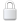Log In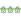Get Password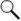Search Archives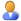Subscribe or Unsubscribe